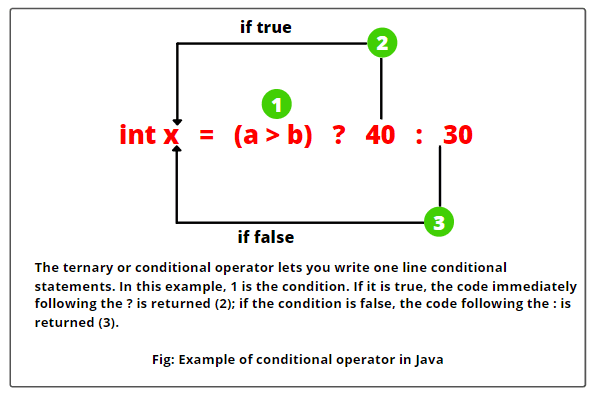# Conditional Operator in Java | Ternary, Example

Conditional operator in Java provides a one line approach for creating a simple conditional statement.

It is often used as a shorthand method for if-else statement. It makes the code much more simple, shorter and readable.

The conditional operator (?:) is also known as ternary operator in java because it takes three operands and perform a conditional test.

The basic syntax to use conditional operator in java program is as follows:

Syntax:

```variable = exp1 ? exp2 : exp3;
where exp1, exp2, and exp3 are expressions.```

## Conditional Operator ? : works as follows:

First of all, the expression exp1 is evaluated. If it is true, the expression exp2 will be evaluated and the value of exp2 will store in the variable. If exp1 is false, exp3 will be evaluated and its value will store in the variable.

Consider the following example:

```int a = 40;
int b = 30;
int x = (a > b) ? a : b;```

Here, first (a > b) is evaluated. If it is true, the value of a is stored in the variable x. If it is false, the value of b is stored in the variable x.

Here, (a > b) is true because the value of a is greater than the value of b. Therefore, the value of a will assign in the variable x. That is, x = 40.In the above example, we have used 3 variables a, b, and x. That’s why it is called ternary operator in java. This example can also be achieved by using the if-else statement as follows:

```if(a > b)
{
x = a;
}
else {
x = b;
}```

Let’s create a program where we will find out the greatest number between the two numbers.

Program code 1:

```package operatorPrograms;
public class Test
{
public static void main(String[] args)
{
int x = 20;
int y = 10;
int z = (x > y) ? x : y;
System.out.println("Greatest number: " +z);
}
}```
```Output:
Greatest number: 20```

2. Let’s create a Java program where we will check that a person is eligible to vote or not.

Program code 2:

```package javaProgram;
import java.util.Scanner;
public class EligibleToVote {
public static void main(String[] args)
{
// Create a Scanner class to accept input from the user.
Scanner sc = new Scanner(System.in);
// Prompt the user to input age for voting.
int age = sc.nextInt();

String str = "You are eligible to vote";
String str2 = "You are not eligible to vote";
String eligible = (age >= 18) ? str : str2;
System.out.println(eligible);
}
}
```
```Output:
25
You are eligible to vote
17
You are not eligible to vote
```

3. Let’s create a Java program using conditional operator to check whether a year is a leap year or not.

Program code 3:

```package javaProgram;
import java.util.Scanner;
public class LeapYearCheck {
public static void main(String[] args)
{
// Create a Scanner class to accept input from the user.
Scanner sc = new Scanner(System.in);
// Prompt the user to input year.
System.out.println("Enter a year: ");

int year = sc.nextInt();
int check4 = year % 4 == 0 ? 1 : 0;
int check100 = year % 100 == 0 ? -1: 0;

int check400 = year % 400 == 0 ? 1 : 0;
int total = check4 + check100 + check400;

String s = "Leap year";
String str = "Not leap year";
String leapYear = total == 1 ? s : str;
System.out.println(leapYear);
}
}
```
```Output:
Enter a year:
2016
Leap year
```

In the preceding example program, we have used the following logic to test leap year. They are as follows:

1. If the entered year is divisible by 4, add 1.
2. If the entered year is divisible by 100, subtract 1.
3. If the entered year is divisible by 400, add 1.
4. If the total is 1, the year is a leap year, otherwise not a leap year.

The general rule to check a leap year is that if a year is divisible by 4, and it is not divisible by 100, unless it is also divisible by 400.

For example, the years 1900 and 2100 are divisible by 100, but not divisible by 400. Therefore, they are not leap year. However, the year 2000 is a leap year.

Try It Yourself

Program code 4:

```public class Test {
public static void main(String[] args)
{
int x = 20;
int y = 20;
++x;
y--;
int z = x < y ? x : y;
System.out.println(z);
}
}
```

Program code 5:

```public class Test {
public static void main(String[] args)
{
int x = 2;
int y = 4;
int z = ++x < y-- - 1 ? x : y;
System.out.println(z);
}
}
```

Program code 6:

```public class Test {
public static void main(String[] args)
{
int x = 2;
int y = 4;
int z = x++/2 == y-- % 3 ? x : y;
System.out.println(z);
}
}
```

In this tutorial, you learned conditional operator (ternary operator) in java with example programs. Hope that we have covered all important points related to the conditional operator  and practice programs based on it.